# Key-finding algorithm

This document describes the Krumhansl-Schmuckler key-finding algorithm. If you have music data that consists of note pitch and duration information, this algorithm can be used to assign an appropriate major or minor key to the music data.

## Correlation coefficient

For a set of (x, y) coordinates, their correlation coefficient is a number from -1 to +1 that indicates if the coordinates have a linear relationship. 0 indicates no linear relationship. +1 indicates a perfectly linear relationship where when one coordinate increases, the other also increases. -1 indicates a perfectly linear relationship where when one coordinate increases, the other decreases.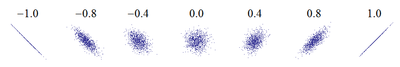For the coordinates (x1, y1) to (xn, yn), the correlation coefficient R is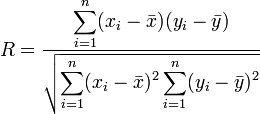where x is the mean of the x coordinates and y is the mean of the y coordinates.

## Key-finding algorithm

In the key-finding algorithm one value of the coordinates represents a profile of a major key or a minor key.

 do do# re re# mi fa fa# so so# la la# ti 6.35 2.23 3.48 2.33 4.38 4.09 2.52 5.19 2.39 3.66 2.29 2.88

 la la# ti do do# re re# mi fa fa# so so# 6.33 2.68 3.52 5.38 2.60 3.53 2.54 4.75 3.98 2.69 3.34 3.17

The other value of the coordinates is the total durations of each pitch class in the piece of music being analyzed.

 C C# D D# E F F# G G# A A# B 432 231 0 405 12 316 4 126 612 0 191 1

The key-finding algorithm calculates a correlation coefficient for each possible major and minor key by pairing the pitch class values to the profile values for the key in question.

 (do , C ) (6.35, 432) (do# , C# ) (2.23, 231) (re , D ) (3.48, 0) (re# , D# ) (2.33, 405) (mi , E ) (4.38, 12) (fa , F ) (4.09, 316) (fa# , F# ) (2.52, 4) (so , G ) (5.19, 126) (so# , G# ) (2.39, 612) (la , A ) (3.66, 0) (la# , A# ) (2.29, 191) (ti , B ) (2.88, 1)R = -0.00009
 (do , C# ) (6.35, 231) (do# , D ) (2.23, 0) (re , D# ) (3.48, 405) (re# , E ) (2.33, 12) (mi , F ) (4.38, 316) (fa , F# ) (4.09, 4) (fa# , G ) (2.52, 126) (so , G# ) (5.19, 612) (so# , A ) (2.39, 0) (la , A# ) (3.66, 191) (la# , B ) (2.29, 1) (ti , C ) (2.88, 432)R = 0.54
 (do , D ) (6.35, 0) (do# , D# ) (2.23, 405) (re , E ) (3.48, 12) (re# , F ) (2.33, 316) (mi , F# ) (4.38, 4) (fa , G ) (4.09, 126) (fa# , G# ) (2.52, 612) (so , A ) (5.19, 0) (so# , A# ) (2.39, 191) (la , B ) (3.66, 1) (la# , C ) (2.29, 432) (ti , C# ) (2.88, 231)R = -0.74
· · ·
 (do , B ) (6.35, 1) (do# , C ) (2.23, 432) (re , C# ) (3.48, 231) (re# , D ) (2.33, 0) (mi , D# ) (4.38, 405) (fa , E ) (4.09, 12) (fa# , F ) (2.52, 316) (so , F# ) (5.19, 4) (so# , G ) (2.39, 126) (la , G# ) (3.66, 612) (la# , A ) (2.29, 0) (ti , A# ) (2.88, 191)R = -0.20
 (la , C ) (6.33, 432) (la# , C# ) (2.68, 231) (ti , D ) (3.52, 0) (do , D# ) (5.38, 405) (do# , E ) (2.60, 12) (re , F ) (3.53, 316) (re# , F# ) (2.54, 4) (mi , G ) (4.75, 126) (fa , G# ) (3.98, 612) (fa# , A ) (2.69, 0) (so , A# ) (3.34, 191) (so# , B ) (3.17, 1)R = 0.62
 (la , C# ) (6.33 , 231) (la# , D ) (2.68, 0) (ti , D# ) (3.52, 405) (do , E ) (5.38, 12) (do# , F ) (2.60, 316) (re , F# ) (3.53, 4) (re# , G ) (2.54, 126) (mi , G# ) (4.75, 612) (fa , A ) (3.98, 0) (fa# , A# ) (2.69, 191) (so , B ) (3.34, 1) (so# , C ) (3.17, 432)R = 0.09
 (la , D ) (6.33 , 0) (la# , D# ) (2.68, 405) (ti , E ) (3.52, 12) (do , F ) (5.38, 316) (do# , F# ) (2.60, 4) (re , G ) (3.53, 126) (re# , G# ) (2.54, 612) (mi , A ) (4.75, 0) (fa , A# ) (3.98, 191) (fa# , B ) (2.69, 1) (so , C ) (3.34, 432) (so# , C# ) (3.17, 231)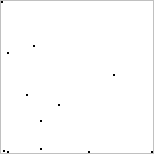R = -0.31
· · ·
 (la , B ) (6.33 , 1) (la# , C ) (2.68, 432) (ti , C# ) (3.52, 231) (do , D ) (5.38, 0) (do# , D# ) (2.60, 405) (re , E ) (3.53, 12) (re# , F ) (2.54, 316) (mi , F# ) (4.75, 4) (fa , G ) (3.98, 126) (fa# , G# ) (2.69, 612) (so , A ) (3.34, 0) (so# , A# ) (3.17, 191)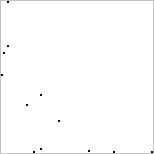R = -0.72

The key with the highest correlation coefficient is used as the key for the piece.

 G# major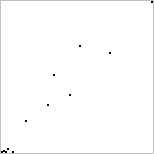R = 0.97

## Where do the profiles come from?

The key-finding method described above is called the Krumhansl-Schmuckler key-finding algorithm (by Carol L. Krumhansl and Mark A. Schmuckler). The profile numbers came from experiments done by Krumhansl and Edward J. Kessler. The experiments consisted of playing a set of context tones or chords, playing a probe tone, and asking a listener to rate how well the probe tone fit with the context. You can read about the experiments and the algorithm in Krumhansl's book Cognitive Foundations of Musical Pitch. (The experiments are described in Chapter 2. The key-finding algorithm is described in Chapter 4.) You may be able to read portions of the book on Google Books.

## Other profiles

The key-finding algorithm can be used with alternative profiles for the major and minor key. Humdrum extras - keycor manpage describes the same key-finding algorithm and lists alternative profiles you can use. The profiles shown on that page are labeled with C major pitches in uppercase for the major profile and C minor pitches in lower case for the minor profile.

 Home > Articles > Key-finding algorithm Robert Hart Posted Aug. 19, 2012